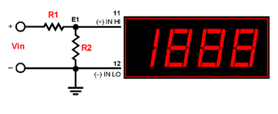# Resistor CalculatorE-96 (±1%) Resistor Decade Values 10.0 10.2 10.5 10.7 11.0 11.3 11.5 11.8 12.1 12.4 12.7 13.0 13.3 13.7 14.0 14.3 14.7 15.0 15.4 15.8 16.2 16.5 16.9 17.4 17.8 18.2 18.7 19.1 19.6 20.0 20.5 21.0 21.5 22.1 22.6 23.2 23.7 24.3 24.9 25.5 26.1 26.7 27.4 28.0 28.7 29.4 30.1 30.9 31.6 32.4 33.2 34.0 34.8 35.7 36.5 37.4 38.3 39.2 40.2 41.2 42.2 43.2 44.2 45.3 46.4 47.5 48.7 49.9 51.1 52.3 53.6 54.9 56.2 57.6 59.0 60.4 61.9 63.4 64.9 66.5 68.1 69.8 71.5 73.2 75.0 76.8 78.7 80.6 82.5 84.5 86.6 88.7 90.9 93.1 95.3 97.6

When measuring voltages greater than the full-scale input range of a given meter, or when the desired display reading is something other than the direct value of the dc input voltage (Vin), the input must be first attenuated before it is presented to the meter's IN LO (-) and IN HI (+) input terminals. A simple two-resistor voltage divider, similar to that shown in the figure above, will scale the input voltage down to the range of the selected meter. For most applications, a +/-2V input meter ("-1" model) is preferred because its high-impedance input will not adversely affect the voltage developed across R2.

Using either the recommended 909K resistor or a user-defined value for R1, the calculator below will quickly determine the correct value for R2. Please note, this calculator program can only be used with +/- 2V ("-1" input range) dc-voltmeters.

Proper calculator operation depends on Vin meeting the following three conditions:

the desired display readings are directly proportional to Vin; a zero volt Vin will display "000" ("0000" for 4½ digit meters); an increase in Vin will result in a corresponding increase in the display reading. Inputs which include either a zero offset or a negative component ("bipolar") will not produce valid R2 values.

All values for R1 and R2 are entered in K Ohms. As a general rule, R1 and R2 should be precision, ±1%, metal-film resistors with absolute TCR's less than 100ppm/°C for 3½ digit meters and less than 50ppm/°C for 4½ digit meters. See the resistor selection chart below and DMS Application Note 4 and Application Note 14 for more information.

## 3½ Digit Resistor Calculator

 DisplayReading R1 Vin E1 ( x k) / ( - Display Reading) = R2 = k Click calculate to run equation or clear to reset input fields.

## 4½ Digit Resistor Calculator

 DisplayReading R1 Vin E1 ( x k) / ( - Display Reading) = R2 = k Click calculate to run equation or clear to reset input fields.

## How to use calculator

 1) Enter the required display reading when the input voltage (Vin) is at its maximum value. If the required display reading includes a decimal point, enter the reading without the decimal point (for example, if the desired display reading is "95.0", enter it as 950). Keep in mind that, disregarding decimal points, 3½ digit meters can display readings up to a maximum of "1999", while 4½ digit meters can display readings up to a maximum of "19999".2) MPS recommends a value for R1 of 909K. However, if desired, R1 values between 50K and 10,000K Ohms can be entered, as long as R1+R2 will not load the input signal.3) Enter the full-scale Vin value, omitting the "V" suffix.4) Click the Calculate button. Using the resulting value for R2, select the nearest 1% resistor value from the chart. IMPORTANT: R1 & R2 voltage and wattage ratings must be chosen to ensure safe and reliable operation under the worst-case applied voltages & currents.
We can't find products matching the selection.